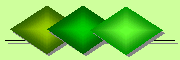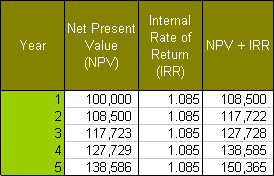De Claron LtdExpress Accountancy services at affordable prices
 Accounting Property Investment Reporting Company Secretarial ServicesHelp

Discounted Cash Flows

Discounted Cash Flows (DCF) is used to value anticipated cash flows from investment properties. In contrast to Capitalisation Rates and Gross Rental Multipliers which tends to be used more for residential properties, DFC values commercial properties such as major office complexes, large shopping centres and industrial parks.

Put simply DCF is an estimate of any future cash flows derived from an investment property. It measures the value of cash flows against time and revises that figures in today's terms. This is called the present discounted value.

The following example will illustrate how to calculate DCF over a specified period:

If a house was bought for \$100,000 and it was anticipated that in 5 years time it would sell for \$150,000 then the expected profit would be \$50,000. To estimate payments for each successive year over the 5 year period these payments would be spread evenly for the running cumulative total of \$150,000. This is done by amortising the internal rate of return (IRR) to about 8.5%.

• 1.0855 x \$100,000 = \$150,000
• The the IRR is a the amount of money received from an investment. It is expressed as a percentage of the money received over the original investment amount. In the abvoe example, if one bought a building for \$100,000 and sold it for \$150,000 in 5 years time then the internal rate of return (IRR) is:

• 50,000 / 100,000 = 50%
• Since, however, payment is received after five years the IRR has to be amortised. That is, the payments are spread evenly over the five year period so the amortised IRR is 8.50% per year. This is illustrated by the amortisation table below:This table shows the IRR expressed as 1.085 which is the principal of \$100,000 at 1.0 and the per annum rate of return on the principal at 0.085 or 8.5%. Payments made on the IRR of 1.085 are done a cumulative basis for each successive year. That is, the IRR is calculated on the running total Net Present Value (NPV) whereby the money received from the principal and the return for the previous year is used to calculate the total money to be received for the next year so repayments go up exponentially.

Time Value of Money
It can be seen from the above that though the house is valued at \$150,000 in 5 years time it is still only worth \$100,000 today. That is, the future value of the house at \$150,000 has been "discounted" to \$100,000 in today's figures. Or in other words, this is the present discounted value for the time value of money of the house.

Cost of Capital
However, the present discounted value is also based on the cost of capital of the house. It goes on the assumption that any cash flows received from an investment property is not worth as much now as their stated future value because of the uncertainty about whether such future values will be actually realised.

DCF places a value on this uncertainty (the risk factor) and correspondingly reduces or "discounts" the future cash flows estimates to the present to what they are maybe worth now. This estimate invovles two steps:

First, we compare the risk of discounting the value of the house to the return from non-risk investments. An example of the latter is government issued bonds which have a guaranteed (i.e. risk-free interest rate) of let's say 2.5% over 5 years. If we choose not to invest the \$100,000 in these risk-free bonds we correspondingly incur the risk that \$100,000 may not realise its expected value in 5 years time when the house is sold. This is expressed by discounting the internal rate of return by 2.5% for the lost opportunity of obtaining risk-free income from the government bonds. That is, we only consider the excess return achieved over the risk-free rate.

• (internal rate of return at 8.5%) - (non-risk factor of 2.5%) = revised internal rate of return at 6.0%
• Second, the internal rate of return will have to be revised further to take into account risk factors whereby the house maybe sold for less than \$150,000 in 5 years time or it may not even be sold at all. Such risk factors encompass declining real estate prices, higher interest rates on mortgages and so forth.

Estimating such risk factors becomes more unreliable over time. However, if we assign a risk factor of 3.0% then this should be deducted from the above revised internal rate of return of 6.0% to give a revised figure of 3.0%.

• internal rate of return at 6.0% - risk factor of 3.0% = revised internal rate of return at 3.0%
• This revised internal rate of return of 3.0% is still greater than the 2.5% interest earned from the risk-free government bonds. However, the difference is only 0.5%

Now we amortise the revised internal rate of return of 3% to give the value of the house in 5 years time.

• 1.035 x \$100,000 = \$115,927
• With this figure we now revise our earlier estimate of the selling price of the house of \$150,000 in 5 years time. Instead it is now anticipated that the house will sell for \$115,927 for an expected profit of \$15,927 instead of \$50,000.

Related topics:

• Net Present Value
• Capitalisation Rates
• Revenue Multipliers